# 数字图像处理基础练习

## 空间域图像处理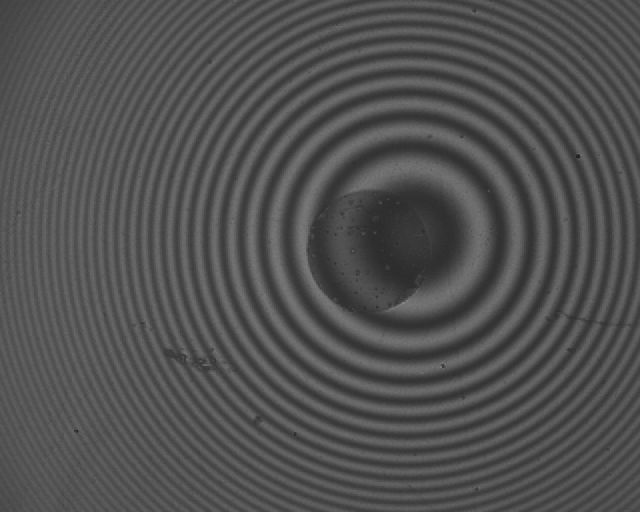◎ 第一幅干涉图◎ 第二幅干涉图

 1 2 3 4 5  a = imread('1.bmp'); b = imread('2.bmp'); pic1 = imlincomb(0.5,a,0.5,b); imshow(pic1); title('加法运算');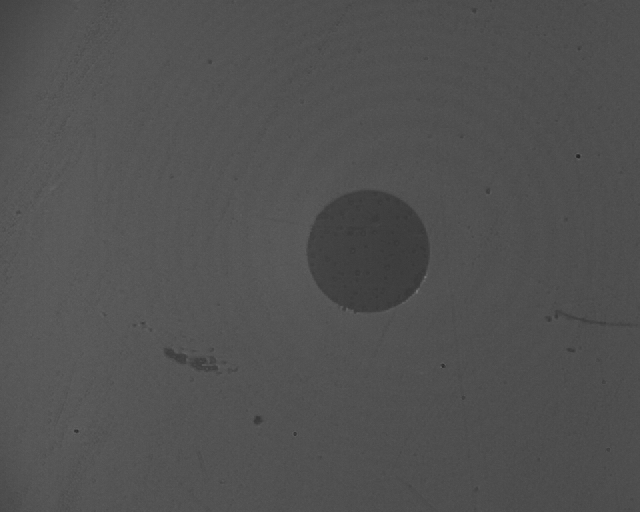◎ 图像相加

 1 2 3  pic2 = imbinarize(pic1,0.29); imshow(pic2); title('二值化');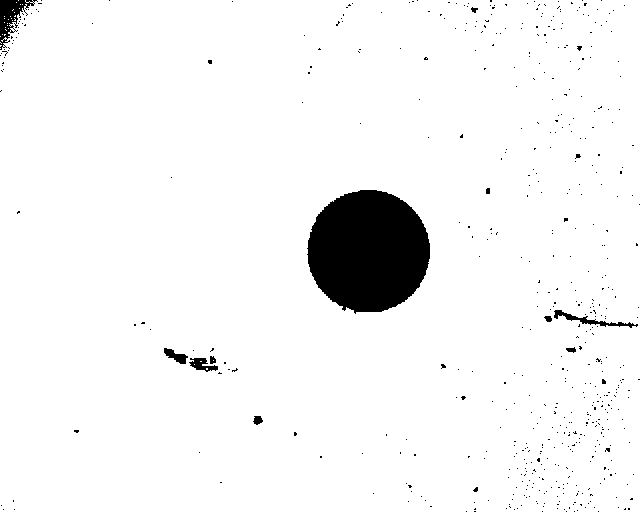◎ 二值化处理

 1 2 3 4 5 6 7  pic3 = edge(pic2,'sobel',0.2); x1 = 280; x2 = 460; y1 = 180; y2 = 320; imshow(pic3(y1:y2,x1:x2)); title('Sobel 算子边缘检测');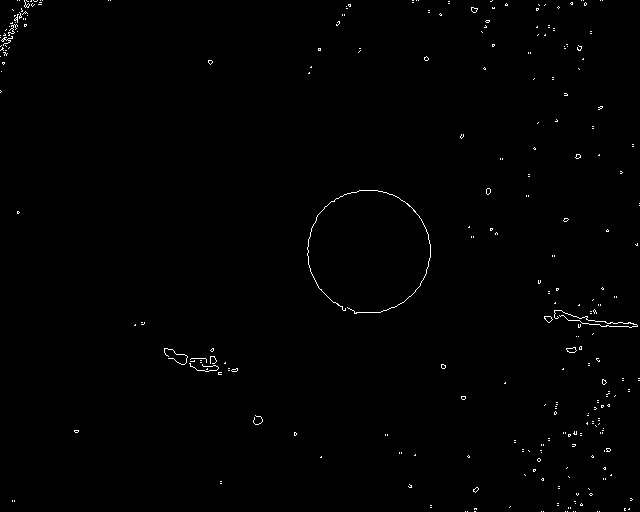◎ Sobel 算子边缘检测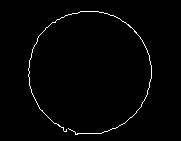◎ 截取后的图像

  1 2 3 4 5 6 7 8 9 10 11 12  pic4 = pic3(y1:y2,x1:x2); [y,x] = find(pic4==1); center_x = min(x)+(max(x)-min(x))/2; center_y = min(y)+(max(y)-min(y))/2; imshow(pic3); hold on; x0 = x1+center_x; y0 = y1+center_y; plot(x0,y0,'*r'); s = sprintf('(%.1f,%.1f)',x0,y0); text(x0,y0,s,'Color','red'); title('标注中心坐标');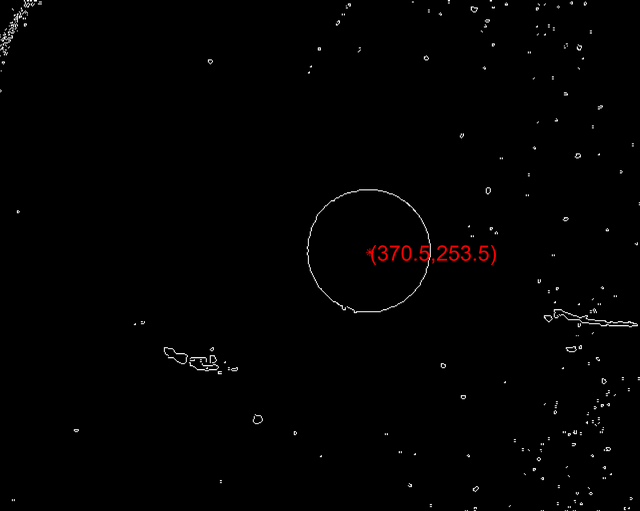◎ 标注坐标

## 频率域图像处理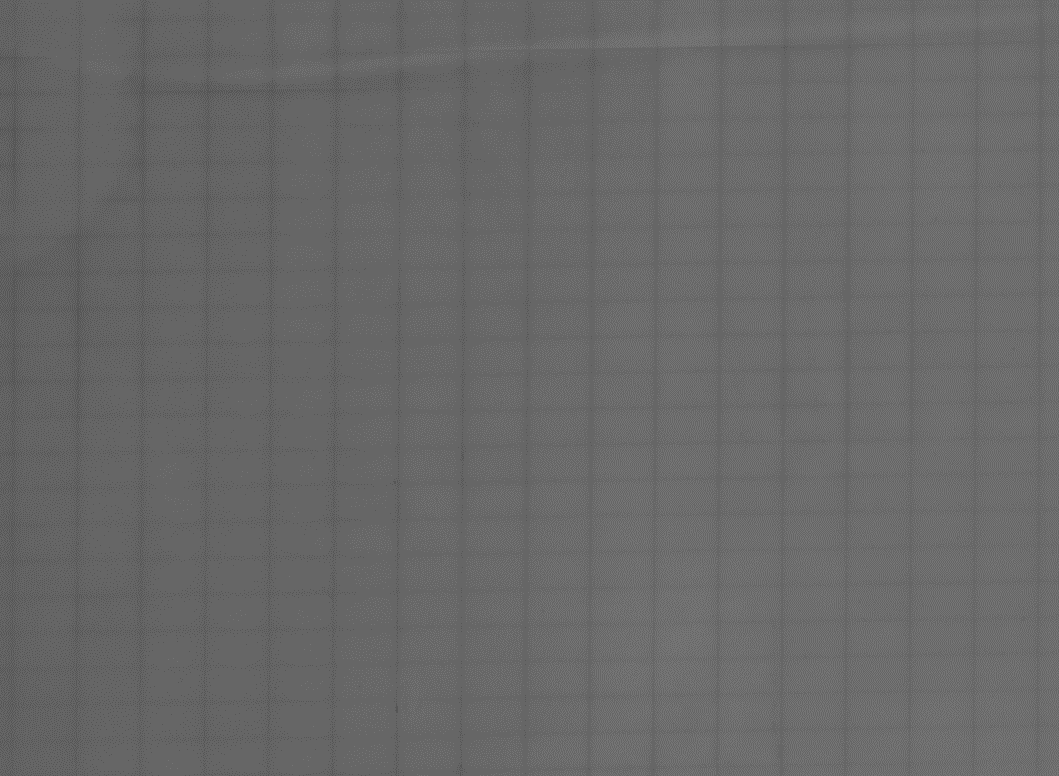◎ 带有周期性干扰的图像

 1 2 3 4 5  I = imread('1.bmp'); I2 = rgb2gray(I); s = fftshift(fft2(I2)); I3 = log(abs(s)); imshow(I3,[]);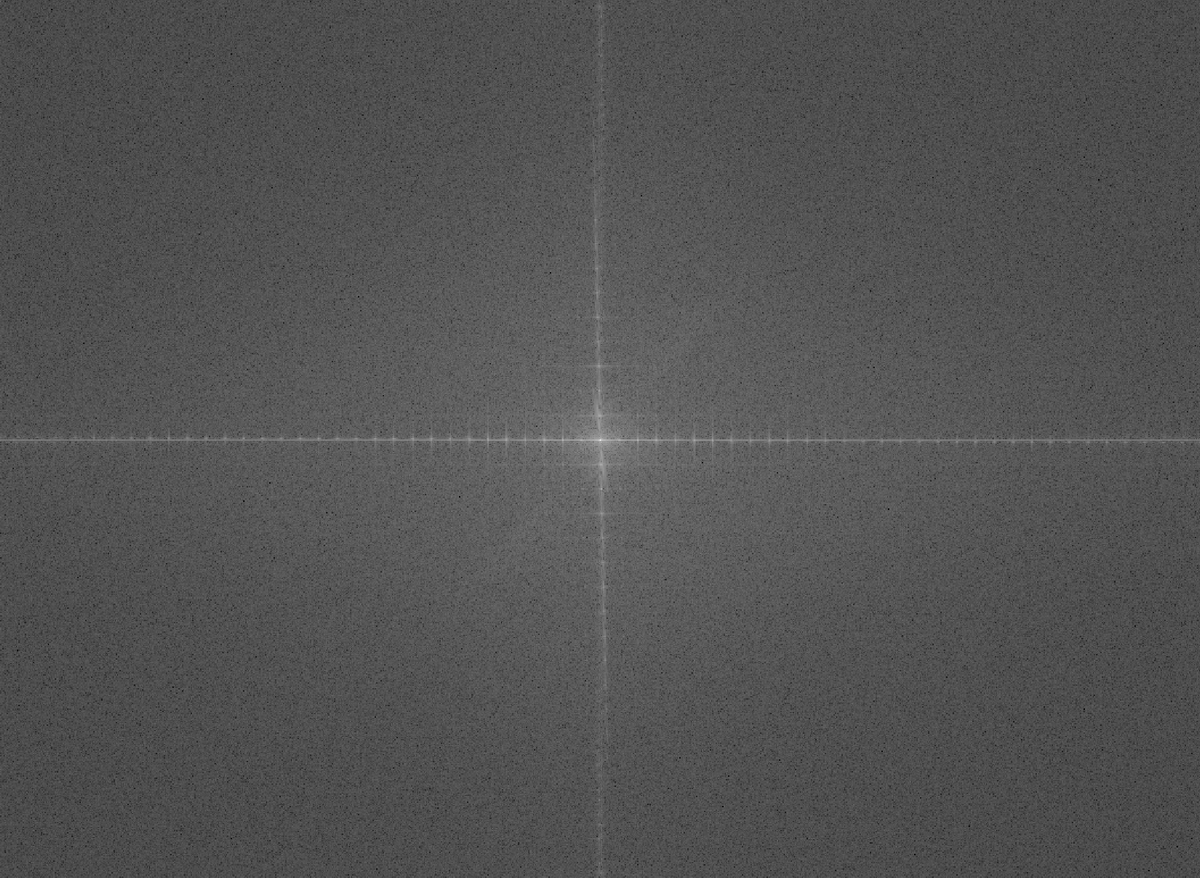◎ 原图的频域图像

  1 2 3 4 5 6 7 8 9 10 11 12 13 14 15 16 17  [a,b] = size(s); a0 = round(a/2); b0 = round(b/2); d = 16; for i = 1:a for j = 1:b distance = sqrt((i-a0)^2+(j-b0)^2); if distance <= d h = 1; else h = 0; end s(i,j) = h.*s(i,j); end end s = uint8(real(ifft2(ifftshift(s)))); imshow(s)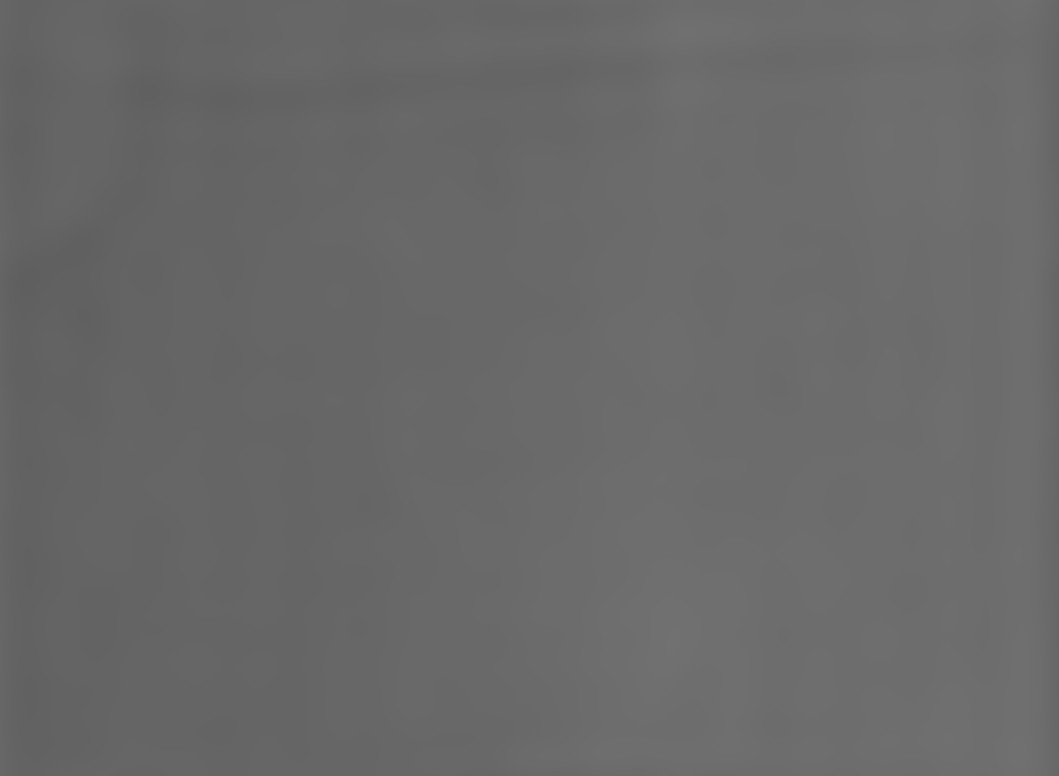◎ 经理想低通滤波器处理后的图像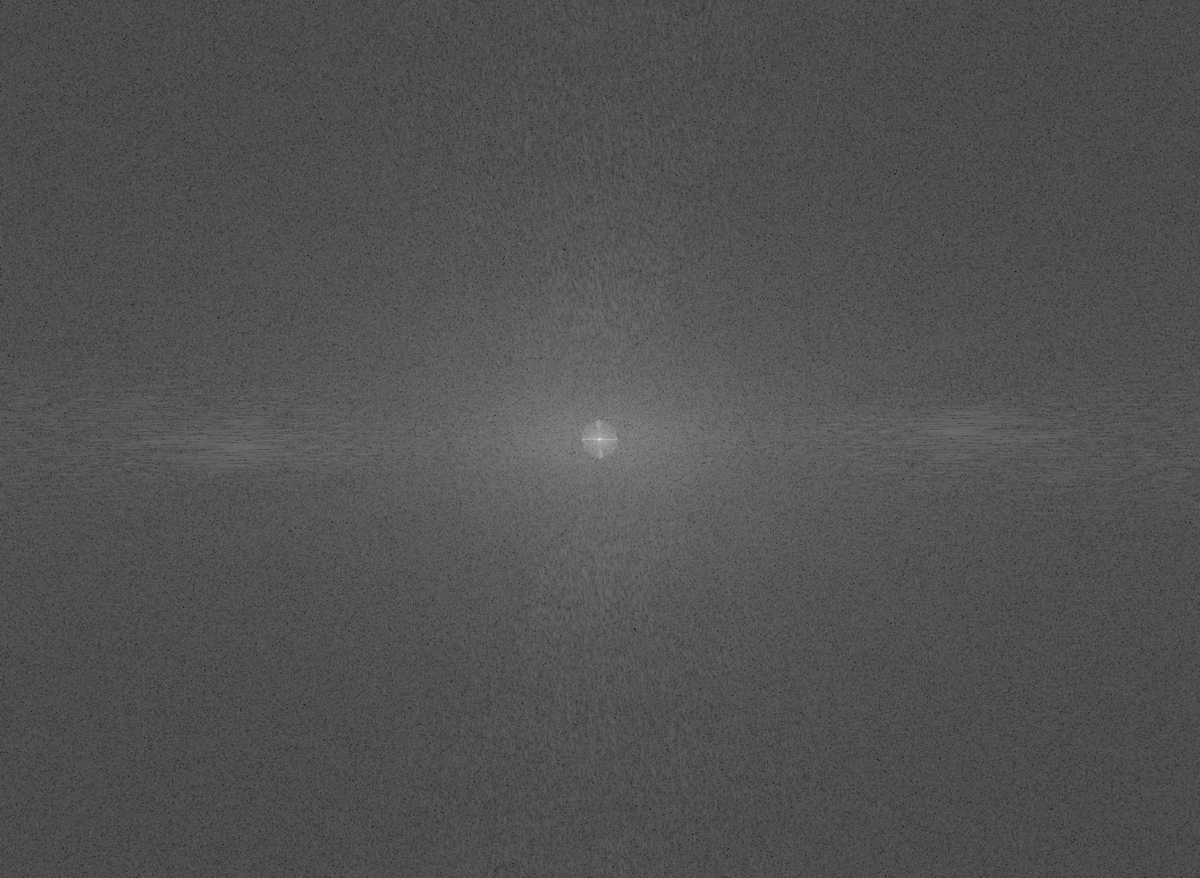◎ 滤波后的频域图像

## 综合练习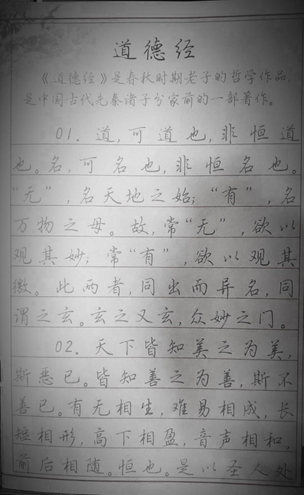◎ 原图带有阴影，文字识别困难

  1 2 3 4 5 6 7 8 9 10 11 12 13 14 15  I1 = imread('img.png'); I2 = rgb2gray(I1); % 转为灰度图 f1 = fspecial('gaussian', [7 7], 0.25); f2 = fspecial('gaussian', [7 7], 1.95); F = f1-f2; %构建一个高通 J1 = log(1.0+double(I2)); %分开入射光和反射光 J2 = imfilter(J1, F, 'symmetric', 'conv'); % 将高通滤波器与对数转换之后的图像卷积 J3 = exp(J2)-1.0; % 变换回来 minJ = min(min(J3)); maxJ = max(max(J3)); J4 = (J3-minJ)/(maxJ-minJ); % 同态滤波 imshow(J4);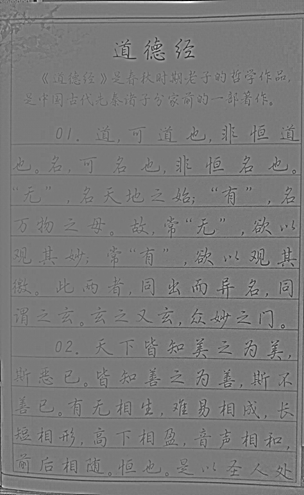◎ 经同态滤波处理后的图像

 1  k = im2bw(J4, 0.462); % 二值化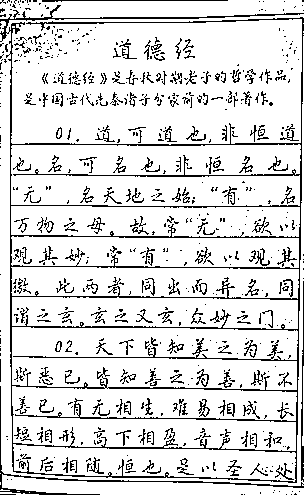◎ 二值化后的图像